# Temperature - math problems

#### Number of problems found: 102

• The level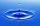The level of temperature of the liquid in a thermometer is 24.32°C lower than the boiling point of water. What is the thermometer reading? Show your answer by drawing and shading the thermometer.
• Temperature up and dowm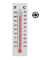At 6 AM, the temperature is 19.4°C. Between 6 AM and noon, the temperature rises 3.8°C. Between noon and 6 PM, the temperature falls 2.5°C. What is the temperature at 6 PM?
• The temperature 13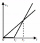The temperature in Toronto at noon during a winter day measured 4°C. The temperature started dropping 2° every hour. Which inequality can be used to find the number of hours, x, after which the temperature will measure below -3°C?
• The temperature 12The temperature of a liquid was 25°C before it was warmed at a rate of 10°C per minute for 5 minutes. It was then cooled at 3.5°C per minute for 6 minutes. What was the temperature of the liquid after cooling?
• Lower temperatureThe level of temperature of the liquid in a thermometer is 24.32°C lower than the boiling point of water. What is the thermometer reading? Show your answer by drawing and shading the thermometer.
• Now isNow is 5°C morning. What will the temperature be if i. It rises by 12°C ii. It falls by 15°C
• ThereThere is 5 l of water with a temperature of 20°C in the bucket. We add 3 liters of hot water. The resulting temperature will be 30°C. What was the temperature of the hot water?
• The temperature 11The temperature on Sunday was 4°C. The temperature dropped 8°C on Monday and twice as much on Tuesday. What is the temperature on Tuesday?
• Thermometer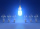Immerse a thermometer with a heat capacity of 2.0 J. K-1 in water weighing 67.0 grams. Before immersion in water, the thermometer showed a temperature of 17.8 degrees Celsius. After reaching equilibrium, the temperature is 32.4 degrees. What was the water
• Latent heat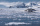How much heat is needed to take from 100 g of water at 20°C to cool to ice at -18°C? Mass heat capacity c (ice) = 21kJ/kg / °C; c (water) = 4.19 KJ/kg / °C and the mass group heat of solidification of water is l = 334kJ/kg
• RoxvilleThe temperature in Roxville decreased 15 degree in five hours. What was the average decrease in temperature per hour? Write you answer as an integer.
• Heat transferWe placed a lead object weighing 0.4 kg and 250°C in 0.4 L water. What was the initial temperature of water t2 if the object's temperature and the water after reaching equilibrium was 35°C? We assume that the heat exchange occurred only between the lead o
• PetraPetra likes to bathe in a bathtub with a water temperature of 38 degrees. She turned on the hot water set at 70 degrees and left for a moment. Before she returned, 40 liters of water flowed into the tub. How much water with a temperature of 22 degrees mus
• At noon 2At noon, the temperature was 4 degrees fahrenheit. At 6pm, it was 10 degrees colder. What was the temperature at 6pm?
• The aluminumThe aluminum object with a weight of 0.80 kg and a temperature of 250°C was placed in the water with a weight of 1.6 kg and a temperature of 23°C. What is the system temperature after reaching equilibrium? We assume that the heat exchange occurred only be
• The bathThe bath has water with a volume of 220 l and a temperature of 65°C. Determine the mass m2 of water at 15°C to be poured so that the resulting temperature of the water in the bath is 40°C. We neglect the heat exchange between water and the environment. T
• If the 3If the temperature of a city drops 2° everyday and increases by 3° on every 5 days, how long does it take to drop to -15° from temperature +5°?
• Ground levelThe temperature at ground level in Helsinki is 20°C lower than in London. The temperature in a basement flat in Helsinki is 5°C below the temperature at ground level. If it is -29°c in the basement, what is the temperature in London?
• At midmorningThe temperature at midmorning was 31°C. Early in the evening, the temperature dropped by 5°C. What was the resulting temperature?
• WeatherWeather. At 5:00 am, the temperature was 28°C, and at noontime, it rose by 9°C. By 4:00 pm, it dropped 3°C, and by nighttime, it was 4°C less. What is the room temperature this time?

Do you have an exciting math question or word problem that you can't solve? Ask a question or post a math problem, and we can try to solve it.

We will send a solution to your e-mail address. Solved examples are also published here. Please enter the e-mail correctly and check whether you don't have a full mailbox.

Please do not submit problems from current active competitions such as Mathematical Olympiad, correspondence seminars etc...